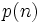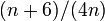# Commuting fraction

## Definition

### In terms of probability of satisfaction of a word

The commuting fraction or commuting probability or commutativity ratio of a finite group is defined as the probability of satisfaction of the commutator as a word. Note that it does not matter which of the conventions (left or right) we choose to define the commutator. With the left convention, the commutator is$xyx^{-1}y^{-1}$, and with the right convention, the commutator is$x^{-1}y^{-1}xy$.

### In terms of fraction of pairs that commute

For a finite group$G$, define:$CP(G) := \{ (x,y) \in G \times G \mid xy = yx \}$

The commuting fraction or commuting probability or commutativity ratio of$G$ is the quotient:$\frac{|CP(G)|}{|G|^2}$

It can also be viewed as the probability that two elements of the group, picked independently uniformly at random, commute.

Note that:$|CP(G)| = \sum_{g \in G} |C_G(g)|$

So the commuting fraction of$G$ can be written as:$\sum_{g \in G} \frac{|C_G(g)|}{|G|^2}$

Note that this can also be written as follows, where$Z(G)$ is the center of$G$:$\sum_{g \in Z(G)} \frac{|C_G(g)|}{|G|^2} + \sum_{g \in G \setminus Z(G)} \frac{|C_G(g)|}{|G|^2}$

This simplifies to:$\frac{|Z(G)|}{|G|} + \sum_{g \in G \setminus Z(G)} \frac{|C_G(g)|}{|G|^2}$

### In terms of number of conjugacy classes

The commuting fraction of a finite group is defined as the quotient of its number of conjugacy classes by its order.

### In terms of index of centralizers

This formulation has the advantage that, in addition to making sense for a finite group, it also makes sense for a FZ-group, i.e., a group whose center has finite index, i.e., the inner automorphism group is a finite group. If the whole group is finite, it agrees with the usual definition.

The commuting fraction is defined as:$\frac{1}{[G:Z(G)]} \sum_{t \in T} \frac{1}{[G:C_G(t)]}$

where$[G:H]$ denotes the index of$H$ in$G$,$Z(G)$ denotes the center of$G$,$T$ is a left transversal for$Z(G)$ in$G$, and$C_G(t)$ is the centralizer of$t$ in$G$. Note that this involves a finite sum of finite numbers, hence it makes sense.

Also, since any two elements in the same coset of$Z(G)$ in$G$ have the same centralizer,$C_G(t)$ depends only on the coset of$t$, and the sum is hence independent of the choice of$T$.

### Equivalence of definitions

Further information: Equivalence of definitions of commuting fraction

The equivalence follows from the orbit-counting theorem.

## Particular cases

Here are the commuting fractions for some non-abelian groups of small order. Note that all abelian groups have commuting fraction equal to$1$, so these are omitted from the list. Note that the commuting fraction is the quotient of the number of conjugacy classes to the order of the group.

Group Order GAP ID second part Number of conjugacy classes Commuting fraction in proper fraction form Commuting fraction in decimal (rounded three places) Comment
symmetric group:S3 6 1 3 1/2 0.500 See cycle type determines conjugacy class and element structure of symmetric groups. The general formula is$p(n)/n!$ where$p(n)$ is the number of unordered integer partitions and is also the number of conjugacy classes.
dihedral group:D8 8 3 5 5/8 0.625 The general formula for$D_{2n}$,$n$ even, is$(n + 6)/(4n)$. See element structure of dihedral groups, element structure of dihedral group:D8, and commuting fraction equals five-eighths iff inner automorphism group is Klein four-group.
quaternion group 8 4 5 5/8 0.625 See commuting fraction equals five-eighths iff inner automorphism group is Klein four-group.
dihedral group:D10 10 1 4 2/5 0.400 The general formula for$D_{2n}$,$n$ odd, is$(n + 3)/(4n)$. See element structure of dihedral groups
dicyclic group:Dic12 12 1 6 1/2 0.500 See element structure of dicyclic groups
alternating group:A4 12 3 4 1/3 0.333 See element structure of alternating groups
dihedral group:D12 12 4 6 1/2 0.500 See element structure of dihedral groups
symmetric group:S4 24 12 5 5/24 0.208 See element structure of symmetric groups